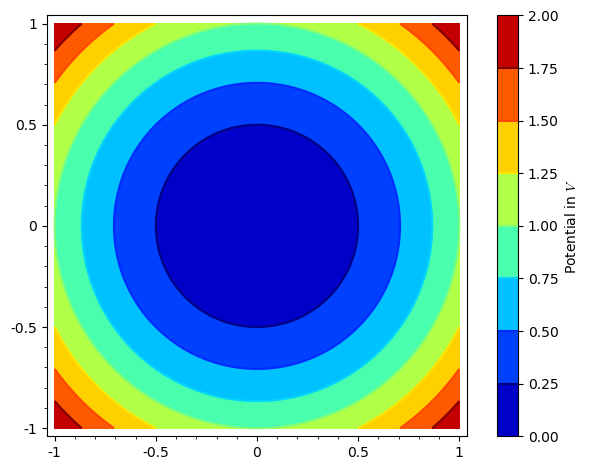# How to label your colorbar in contour plots?

i looked at the options of the contour_plot funciton and tried this:

contour_plot(pic, (x,-1,1), (z,-1,1), region=region_to_include, contours= 30, plot_points=200, cmap=colormaps.jet, colorbar=True, axes_labels=['$x$ in [m]','$z$ in [m]'], legend_label=['Potential in $V$'])


but that does nto give any effect.

I want to put the unit of my plot there. how can i do that?

edit retag close merge delete

Sort by » oldest newest most voted

Hello. What I understand is that you want to put "Potential in $V$" as your colorbar legend (?). I have a little complicated form, but I think it will help you. Let me give you an example smaller than your plot:

var('y')
p = contour_plot(x^2+y^2,(x,-1,1), (y,-1,1), cmap=colormaps.jet, colorbar=True)


Now you have stored you contour plot inside the variable p. This variable actually is a list of plots (in this case, it just contains your single plot in p, but if you were to make something like p+=plot(x^2), then this second graphic would be stores in p, and so on). Let's separate your plot in another variable; you do this with:

plt = p


Now you can access all the options of your plot. For example, if you type:

opt = plt.options()
print(opt)


you will see there is a part labeled "colorbar_options"; if you don't see it, you can just replace the last line with

opt['colorbar_options']


This is a Python dict. We will add a field called "label" which will be the label of your colorbar. In this case:

opt['colorbar_options']['label'] = 'Potential in $V$'


That's it. Now you have to do the same steps backwards to apply the new options:

plt.set_options(opt)
p = plt


Finally, you plot it:

p.show()


The result should be like this:You can also check the whole code in this Sage Cell page: Contour plot with labelled colorbar

I'm sorry I didn't find another easier way to do this. However, you can wrap this code in a function (if you can't do it, let me know so I can send it to you). If I find another way, I'll write it.

I hope this helps!

more